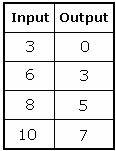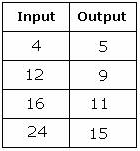Definition Of Rule

Row Matrix is a matrix with only one row.

Example of Rule

7, 16, 43,... is the pattern for which the rule is "Multiply by 3 and subtract by 5 to get the next number" as each number is obtained by multiplying by 3 and then subtracting the result by 5 to get the next numberThe first value in the input column is 3 and the output is 0. This is obtained by adding 2 to the input value and subtracting 5 from the result, i.e. 3 + 2 = 5 - 5 = 0.
The second value in the input column is 6 and the output is 3. This is obtained by adding 2 to the input value and subtracting 5 from the resultant value, i.e. 3 + 2 = 5 - 5 = 0
The third value in the input column is 8 and the output is 5. This is obtained by adding 2 to the input value and subtracting 5 from the resultant value, i.e. 8 + 2 = 10 - 5 = 5
The fourth value in the input column is 10 and the output is 7. This is obtained by adding 2 to the input value and subtracting 5 from the resultant value, i.e. 10 + 2 = 12 - 5 = 7.

Solved Example on Rule

Ques: What is the rule for the table?Choices:

A. Add 2 and multiply by 1
B. Multiply by 2 and subtract 2
C. Subtract 2 and add 4
D. Divide by 2 and add 3